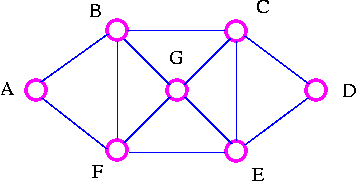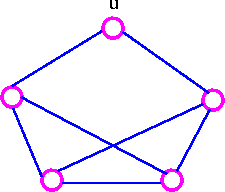Graph Theory

## Euler GraphsThe explorer's Problem: An explorer wants to explore all the routes between a number of cities. Can a tour be found which traverses each route only once? Particularly, find a tour which starts at A, goes along each road exactly once, and ends back at A.

Examples of such tour are

 A B C D E F B G C E G F A A F G C D E G B C E F B A

The Explorer travels along each road (edges) just once but may visit a particular city (vertex) several times.

The Traveler's Problem

A traveler wants to visit a number of cities. Can a tour be found which visits each city only once? Particularly, find a tour which starts at A, goes to each city exactly once, and ends back at A.

Examples of such tour are

 A B C D E G F A A F E D C G B A

The travelers visits each city (vertex)  just once but may omit several of the roads (edges) on the way.

Eulerian Trail

A connected graph G is Eulerian if there is a closed trail which includes every edge of G,  such a trail is called an Eulerian trail.

Hamiltonian Cycle

A connected graph G is Hamiltonian if there is a cycle which includes every vertex of G; such a cycle is called a Hamiltonian cycle.

Consider the following examples:This graph is BOTH Eulerian and Hamiltonian.This graph is Eulerian, but NOT Hamiltonian.This graph is an Hamiltionian, but NOT Eulerian.This graph is NEITHER Eulerian NOR Hamiltionian

Theorem     Let G be a connected graph. Then G is Eulerian if and only if every vertex of G has even degree.

• Necessary Condition
• If G is Eulerian, then every vertex of G has even degree.
• Sufficient Condition
• If every vertex of G has even degree, then G is Eulerian.

Eulerian-Type Problems

• Edge-traceable graphs.
• Diagrams-Tracing Puzzles.
• Dominoes.
• Mazes and labyrinths,
• The Chinese Postman Problem.
• The Rotating Drum Problem.

Neither necessary nor sufficient condition is known for a graph to be Hamiltonian. The search for necessary or sufficient conditions is a major area of study in graph theory today.

Sufficient Condition

Dirac's Theorem    Let G be a simple graph with n vertices where n ≥ 3 If deg(v) ≥ 1/2 n for each vertex v, then G is Hamiltonian.

For example,n = 6 and deg(v) = 3 for each vertex, so this graph is Hamiltonian by Dirac's theorem.

Ore's Theorem    Let G be a simple graph with n vertices where n ≥ 2 if deg(v) + deg(w) ≥ n for each pair of non-adjacent vertices v and w, then G is Hamiltonian.

For example,n = 5 but deg(u) = 2, so Dirac's theorem does not apply. However, deg(v) + deg(w) ≥ 5 for all pairs of vertices v and w (infact, for all pairs of vertices v and w), so this graph is Hamiltonian by Ore's theorem.

Note that if deg(v) ≥ 1/2 n for each vertex, then deg(v) + deg(w) ≥ n for each pair of vertices v and w. It follows that Dirac's theorem can be deduced from Ore's theorem, so we prove only Ore's threoem.

Hamiltonain - Type Problem

• Gray Codes.
• The Traveling Salesperson Problem
• Ranking in Tournaments.